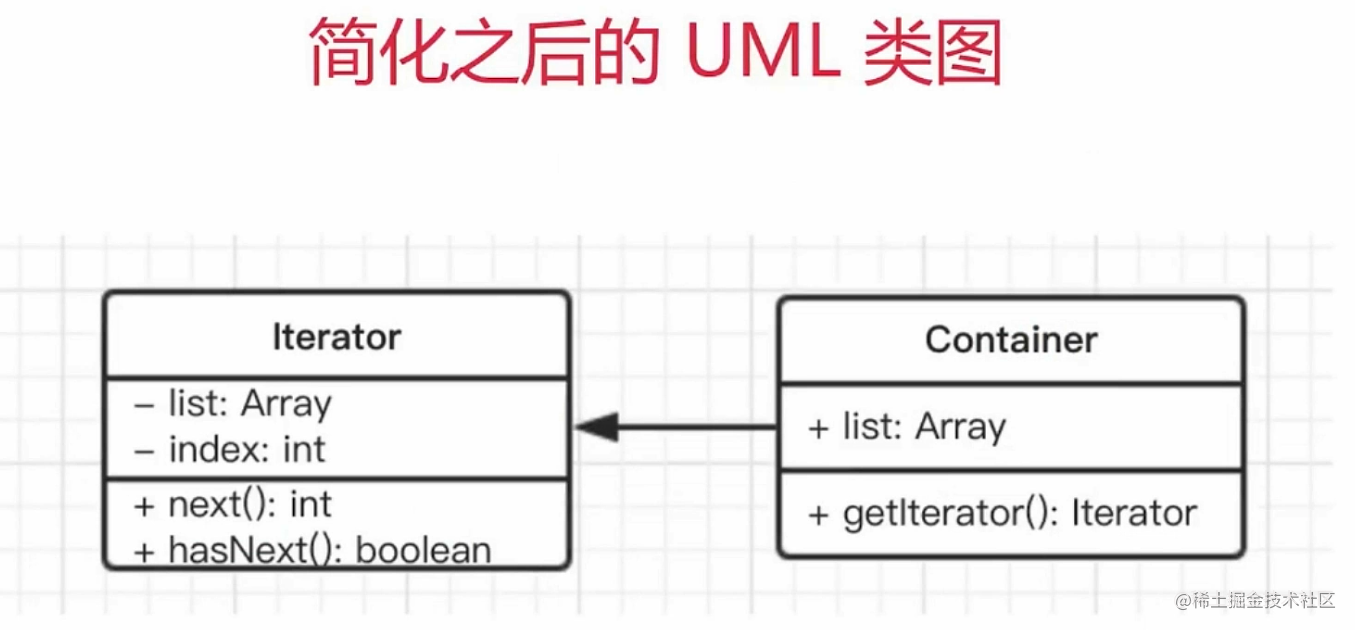# 设计模式之迭代器模式在前端的应用

## 迭代器模式

• 迭代(iterate) - 按顺序访问线性结构中的每一项（只能顺序依次访问）；
• 遍历(traversal) - 按规则访问非线性结构中的每一项（可访问其中一段区间的元素）；

### 基础ES6实现迭代器的例子：

``````class Container {
constructor(list){
this.list = list;
}

getIterator() {
return new Iterator(this);
}
}

class Iterator {
constructor(container){
this.list = container.list;
this.index = 0;
}

next(){
let done = this.index >= this.list.length;

let res = {
value: this.list[this.index],
done: done
};

if(!done) {
this.index++;
}

return res;
}
}

// 测试代码
let arr = [5,6,9,88];
let container = new Container(arr);
let it = container.getIterator();

let tmp = it.next();
while(!tmp.done){
console.log(tmp.value);
tmp = it.next();
}

### 实现目标

• 实现自己的迭代器，把集合中的元素都迭代出来；
• 自己实现的迭代器要支持for(let item of iterator);
``````// 迭代器只要把集合中的元素都迭代出来就行；
// 迭代器不只可以迭代出来元素，还要支持下面的语法
for(let item of iterator){
...
}

#### 小目标1

``````// 有序集合
class ListNode {
constructor(val){
this.val = val;
this.next = null;
}
}

// 2-6-8-5
let head = new ListNode(2);
let n1 = new ListNode(6);
let n2 = new ListNode(8);
let n3 = new ListNode(5);
n1.next = n2;
n2.next = n3;

class Container {
constructor(list){
this.list = list;
}

getIterator(){
return new Iterator(this);
}
}

class Iterator{
constructor(container){
this.list = container.list;
this.prev = this.list;
}

next(){
let done = (this.prev == null);

let res = {
value: done ? null : this.prev.val,
done
};

if(!done) {
this.prev = this.prev.next;
}

return res;
}
}

let container = new Container(head);
let it = container.getIterator();

let temp = it.next();
while(!temp.done){
console.log(temp.value);
temp = it.next();
}

#### 小目标2

``````let obj = {
name: 'David',
age: 20,
[Symbol('my symbol')]: 666
};
console.log(obj, Object.keys(obj));

``````let pro = Object.getOwnPropertySymbols(arr.__proto__);
let it = arr[pro]();

let tmp = it.next();
while (!tmp.done) {
console.log(tmp.value);
tmp = it.next();
}

// 也可以用for...of
for (let item of it) {
console.log(item);
}

``````class Iterator {
constructor(container) {
this.list = container.list;
this.prev = this.list;
}

[Symbol.iterator]() {
let _this = this;
return {
next() {
let done = (_this.prev == null);

let res = {
value: done ? null : _this.prev.val,
done
};

if (!done) {
_this.prev = _this.prev.next;
}

return res;
}
};
}
}

let container = new Container(head);
let it = container.getIterator();

for (let item of it) {
console.log(item);
}